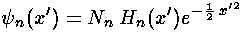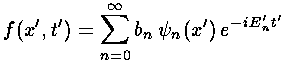## SHM p.7

### Some Details on QM Oscillation

As previously stated, energy eigenfunctions have position probabilities that do not depend on time (although the energy eigenfunctions themselves do have a [complex] time dependence). Thus to see motion (i.e., positions that depend on time), we must not be in a energy eigenfunction. The alternative is to be in several energy eigenfunctions, i.e., to be in a superposition of energy eigenfunctions. Thus the idea is to create a superposition of energy eigenfunctions which initially has a narrow range of position possibilities, and watch that range of position possibilities move.

The game then is a version of Fourier analysis: producing a sum-of-eigenfunctions that adds up to a given function. Orthogonality is what makes this easy; in particular, that our energy eigenfunctions are mutually orthogonal.

Our normalized energy eigenfunctions are gaussian-damped Hermite polynomials:where Nn is given by:These eigenfunctions are orthogonal:So if we try to express a given function f(x') in terms of a sum of these functions:orthogonality allows us to solve for the bn:In this particular example, I choose f(x') to have the shape of the n=52 state, but with f(x')=0 for x'<~9.4 (The first zero of the wavefunction is at ~9.4, thus this f(x') is the solution to the Schrödinger's equation with a quadratic potential for x'>~9.4 and an infinite potential for x'<~9.4.) Of course, we can't sum all the terms of the infinite sum. I've limited the sum to the 17 eigenfunctions near the n=52 eigenfunction. Thus the sum using these 17 bn does not exactly equal the initial f(x'). The below plot displays (in black) f(x') and (in red) the sum using these 17 bn.The missing terms would contribute only 5% additional probability, so it's a fair approximation. To see how this probability lump moves with time, we need only use the time dependence of the energy eigenfunctions:The previous page shows the results: the lump of probability moves just like a classical particle (with broadening of the lump caused by Heisenberg-required uncertainty in initial velocity).